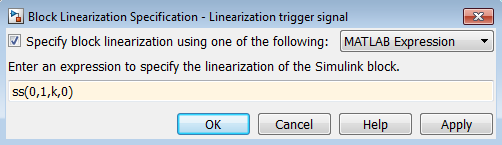Specify Linear System for Block Linearization Using MATLAB Expression

This example shows how to specify the linearization of any block, subsystem, or model reference without having to replace this block in your Simulink® model.

1. Right-click the block in the model, and select Linear Analysis > Specify Selected Block Linearization.

The Block Linearization Specification dialog box opens.

2. In the Specify block linearization using one of the following list, select MATLAB Expression.

3. In the text field, enter an expression that specifies the linearization.

For example, specify the linearization as an integrator with a gain of k, G(s) = k/s.

In state-space form, this transfer function corresponds to ss(0,1,k,0).Click OK.

4. Linearize the model.

Related Topics